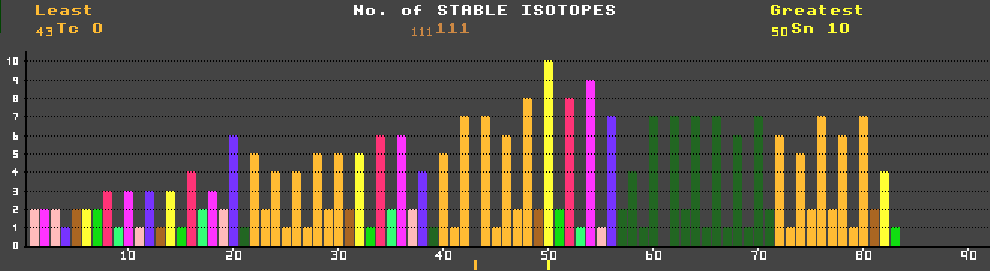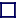### NUMBER of STABLE ISOTOPES per ELEMENT

The isotopes of an element have the same number of protons (or it would not be the same element) and differing numbers of neutrons. Hence the mass number (the number of protons plus neutrons, sometimes shown thus: He-4) is different.

Isotopes are either unstable and radioactive, or stable. Hydrogen (the simplest element with just one proton) has three known isotopes: H-1 (protium) with no neutrons, H-2 (deuterium) with one neutron, and radioactive H-3 (tritium) with two neutrons. Isotopes with too few neutrons are unstable, as are those with too many neutrons; only those with about the right number of neutrons (with about a 1:1 proton:neutron ratio for the light elements) are stable. Heavier elements require proportionately more neutrons for stability. Elements heavier than bismuth (atomic number 83) have no stable isotopes, probably as a result of the accumulative effect of proton-proton repulsion. Note the striking dependence for stability upon the number of protons; those elements with an even number of protons have many more stable isotopes than those with an odd number. Technetium, with an odd number of protons (43), is the lightest element for which no stable isotopes exist. Promethium (61) is next. Tin has the greatest number of stable isotopes at 10, followed closely by xenon, tellurium and cadmium.

The number of neutrons also has an effect (but smaller) on stability; those isotopes with an even number of neutrons are more likely to be stable than those with and odd number. There are only four stable isotopes with odd:odd proton:neutron ratios corresponding to integral spin, whereas there are 165 with even:even (zero spin), and 50 each for odd:even or even:odd (half-integral spin). Those isotopes with a magic number (2, 8, 20, 28, 50, 82 and 126) of either protons or neutrons are much more likely to be stable than others. Thus He-4 (Z=N=2), O-16 (Z=N=8), Ca-40 (Z=N=20), Ni-60 (Z=28), Sr-88 (N=50), Sn-118 (Z=50), Ba-138 (N=82) and Pb-208 (Z=82 and N=126) are particularly stable and have very low neutron absorption csa.